Creating musical sounds

This free course is available to start right now. Review the full course description and key learning outcomes and create an account and enrol if you want a free statement of participation.

Free course

# 5.5 Vibrating string: pitches of notes produced by stringed instruments

When a string is bowed, plucked or struck, energy is supplied that starts the string vibrating. The string doesn't just vibrate in one single mode; instead, it vibrates in a combination of several modes simultaneously. The displacement along the string is the superposition of the standing-wave patterns corresponding to those modes. For example, if the string vibrated only in the first and second modes, the displacement at a given instant of time might appear as shown in Figure 11.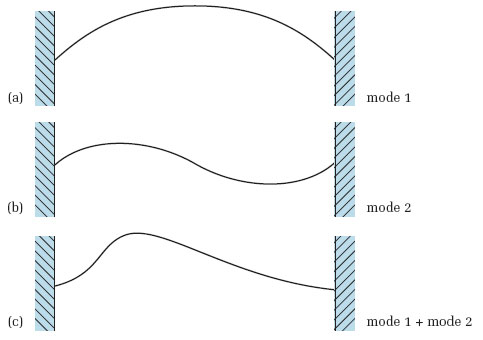Figure 11 Example of the superposition of two waves on a string. The displacement shown in (c) results from adding together, at every point along the string, the displacements shown in (a) and (b)

In instruments such as the violin, guitar and piano, where the string is the primary vibrator, the frequencies at which the instrument vibrates strongly are essentially the natural frequencies of the string. Exciting the string causes both it and the instrument as a whole to vibrate at a number of these natural frequencies simultaneously. Because the natural frequencies of the string are harmonically related, the sound radiated by the instrument will be the sum of a number of harmonics. This will be interpreted by the ear as a single musical note with a pitch corresponding to the fundamental frequency of these harmonics. It is then the relative magnitudes of the harmonic components that provide the basis for the timbre of the note.

To adjust the pitch of notes produced by instruments like the violin, guitar and piano, the string's fundamental frequency must be altered. You learned in Section 5.4 that the fundamental frequency of a string is f1 = v/2L, where v is the speed of a wave travelling on the string and L is the string's length. You also know that the speed of a wave travelling on a string is: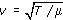where T is the string's tension and μ is its mass per unit length. By combining these two equations, the following expression for the fundamental frequency of a string can be written: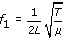Clearly then, to adjust this frequency and hence the pitch of the note produced by the instrument, either the length, tension or mass per unit length of the string must be altered.

You don't have to look very far to find some examples. When playing a guitar, the player presses a finger on a given string to effectively change its length and enable notes of different pitches to be produced. The shorter the effective length of the string, the higher the pitch of the note produced. Alternatively, when tuning a violin, the player tightens or loosens the strings to alter the tension. Increasing the tension causes the note produced to be higher in pitch. Decreasing the tension lowers the pitch of the note produced. As a final example, to be able to achieve the low pitch of the bass strings found on pianos and bass guitars, the core string is wound with additional wire to increase the mass per unit length.

The equation for the fundamental frequency of a string can be rearranged to give the following additional three equations. These equations can be used to find the length, the tension or the mass per unit length required for a string to produce a note of a given pitch: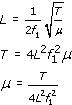You are not expected to memorise these equations, but you do need to be able to use them. One important thing to remember is that when entering values into the equations they must be expressed in SI units: that is, the length must be expressed in metres, the mass per unit length in kilograms per metre and the tension in newtons.

## Activity 15

Suppose the A-string of a violin has been tightened until its tension is 53 N. Its length is 330 mm and its mass per unit length is 0.6 g/m.

1. Calculate the fundamental frequency of the string.

2. The present international pitch standard has the pitch A4 set to a frequency of 440 Hz. Is the instrument in tune, or will it play flat or sharp?

3. Will the string need to be loosened or tightened further to get it in tune?

4. What tension should the string be under?

### Comment

1. The length of the string is given as 330 mm. However, the length must be expressed in metres. Hence, the length of the string L is 330/1000 = 0.33 m. Similarly, the string's mass per unit length is given as 0.6 g/m. This must be expressed in kilograms per metre. Hence, the mass per unit length μ = 0.6/1000 = 0.0006 kg/m. The tension T = 53 N.

Using the equation for f1 given above, the fundamental frequency is then given by: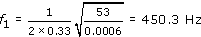2. The fundamental frequency of the string is higher than the international pitch standard of A4 = 440 Hz. Therefore, the instrument will play slightly sharp.

3. To lower the pitch of the note, the tension in the string will need to be reduced. That is, the string will need to be loosened.

4. The tension required for the string to have a fundamental frequency of 440 Hz can be found using the equation for T given above, as follows: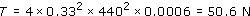## Activity 16

(a) One type of steel piano wire has a mass per unit length of 0.0059 kg/m. If it is under a tension of 700 N, calculate the length of wire required for the string to produce a pitch of C1 (the lowest C on the piano keyboard, with a fundamental frequency of 32.7 Hz).

(b) Your answer to part (a) should have shown you that the length of the wire required is clearly too long to fit in the body of a piano. To reduce the length of wire required to produce a pitch of C1, its mass per unit length is therefore increased by winding extra copper wire around it. Assuming now that the length of a C1 piano string is 1.4 m but it still experiences 700 N of tension, calculate the required mass per unit length if it is to play in tune (remember that the fundamental frequency of C1 is 32.7 Hz).

### Answer

1. (a) The length of wire required for the string to produce a pitch of C1 (fundamental frequency f1 = 32.7 Hz) if its tension is 700 N and mass per unit length is 0.0059 kg/m is: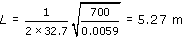2. (b) The mass per unit length required for the 1.4 m long C1 string is: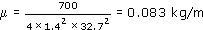TA212_2

### Take your learning further

Making the decision to study can be a big step, which is why you'll want a trusted University. The Open University has 50 years’ experience delivering flexible learning and 170,000 students are studying with us right now. Take a look at all Open University courses.

If you are new to university level study, find out more about the types of qualifications we offer, including our entry level Access courses and Certificates.

Not ready for University study then browse over 900 free courses on OpenLearn and sign up to our newsletter to hear about new free courses as they are released.

Every year, thousands of students decide to study with The Open University. With over 120 qualifications, we’ve got the right course for you.

Request an Open University prospectus